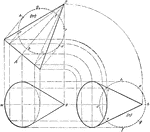Projection Of Cone

Illustration of the projection of a cone that is rightly inclined.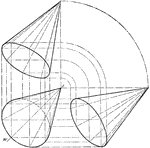Projection Of Cone

Illustration of the projection of a cone that is obliquely inclined.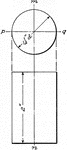Projection Of Cylinder

Illustration of the projection of a cylinder.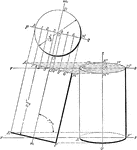Projection Of Cylinder

Illustration of the projection of a cylinder whose axis is parallel to the plane of the paper and makes…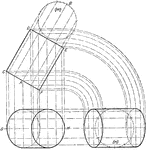Projection Of Cylinder

Illustration of the projection of a cylinder that is rightly inclined.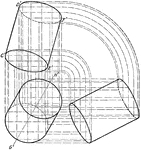Projection Of Cylinder

Illustration of the projection of a cylinder that is obliquely inclined.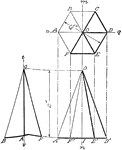Projection Of Hexagonal Prism

Illustration of the projection of a hexagonal prism.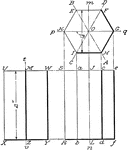Projection Of Hexagonal Prism

Illustration of the projection of a hexagonal prism.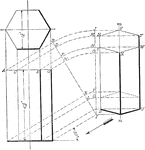Projection Of Hexagonal Prism

Illustration of the projection of a hexagonal prism having two of its parallel sides parallel to the…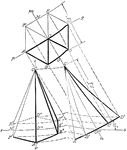Projection Of Hexagonal Prism

Illustration of the projection of a hexagonal prism whose axis is parallel to the plane of the paper…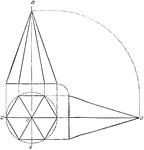Projection Of Hexagonal Pyramid

Illustration of the projection of a hexagonal pyramid that is in a right position.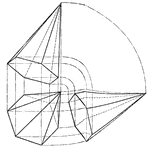Projection Of Hexagonal Pyramid

Illustration of the projection of a hexagonal pyramid that is obliquely inclined.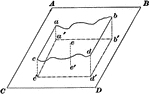Projection Of An Irregular Surface

Illustration of an irregular surface abdc projected upon the flat surface ABCD.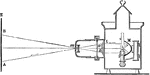Optical Lantern

"The optical lantern is an instrument for projecting on a screen magnified images of transparent photographs,…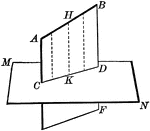Line Projected Upon Plane

Illustration of a straight line projected upon a plane.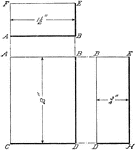Projection Of Rectangular Prism

Illustration of the projection of a rectangular prism that is represented as if it were standing on…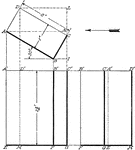Projection Of Rectangular Prism

Illustration of the projection of a rectangular prism whose broad side makes an angle of 30° with…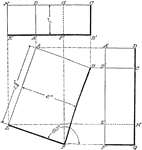Projection Of Rectangular Prism

Illustration of the projection of a rectangular prism whose base makes an angle of 160° with the…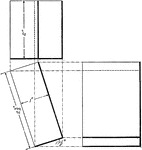Projection Of Rectangular Prism

Illustration of the projection of a rectangular prism whose base makes an angle of 17.5° with the…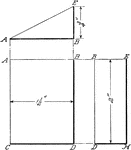Projection Of Wedge

Illustration of the projection of a wedge of a rectangular prism that is standing on one of its triangular…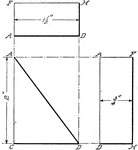Projection Of Wedge

Illustration of the projection of a wedge of a rectangular prism that is standing on one of its rectangular…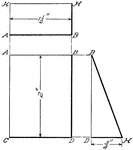Projection Of Wedge

Illustration of the projection of a wedge of a rectangular prism that is viewed from the side.